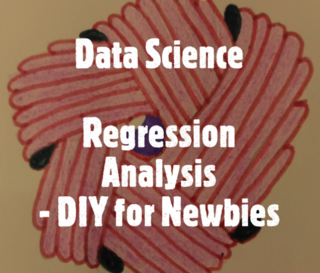\$7.99
Minimum price
\$7.99
Suggested price

### Data Science - Regression Analysis (DIY for newbies)

###### Includes 100 solved problems

The book contains stepwise solutions to regression problems for beginners. In this book, regression concepts are broken down into simple steps. Each problem addresses a concept in regression. The problems are solved using both Python and R programming language.

• ### Categories

• Machine Learning
• Python
• R
• ### Feedback

Email the Author(s)

• Supervised Learning Algorithms:
• Regression
• Polynomial Regression
• Support Vector Regression
• Decision Tree Regression:
• Random Forest Regression:
• 100 Solved Questions
• Q1 (Python - Prediction with Linear Regression)
• Q2 (Python - Linear Regression, PCA, MSE)
• Q3 (R - Prediction with Linear model)
• Q4 (Python - Jaccod Index)
• Q5 (R - Stochastic Gradient Boosting)
• Q6 (Python - Boxplot)
• Q7 (Python - Sort using attrgetter)
• Q8 (Python - Heatmap)
• Q9 (Python - Euclidean Distance)
• Q10 (Python - Manhattan Distance)
• Q11 (R - Bar Plot)
• Q12 (R - Formatting Date)
• Q13 (Python - Forward selection)
• Q14 - (Linear Model)
• Q15 (Python - Correlation)
• Q16 (Python - Manhattan Distance)
• Q17 (Python - Word Split)
• Q18 (Python - PCA)
• Q19 (Python - Eigenvector)
• Q20 (Python - Eigenvalues)
• Q21 (R - Linear Regression)
• Q22 (Python - OLS)
• Q23 (Python - OLS)
• Q24 (Python - Decision Tree)
• Q25 (R - Random Forest)
• Q26 (Python - Text)
• Q27 (Python - Sort)
• Q28 (Python - Indexing)
• Q29 (Python - OLS )
• Q30 (Python - OLS)
• Q31 (Python - Chi-Square)
• Q32 (Python - PCA)
• Q33 (Python - Variance Inflation Factor)
• Q34 (Python - Slope and P-Value)
• Q35 (Python - Recusive Feature)
• Q36 (R - Random Forest)
• Q37 (R - Support Vector Regression)
• Q38 (R - Polynomial Regression)
• Q39 (R - Linear Regression)
• Q40 (Python - Chi value)
• Q41 (R - Attribute Importance)
• Q42 (R - Baggging model)
• Q43 (R - Stacking Algorithm)
• Q44 (R - PCA)
• Q45 (Python)
• Q46 (Python - Random Forest)
• Q47 (R - Forward selection)
• Q48 (R - Support Vector Regressor)
• Q49 (R - Polynomial Regression)
• Q50 (R - Mean, Median, Mode)
• Q51 (Python - Correlation coefficients)
• Q52 (R - Linear Regression)
• Q53 (R - Linear Regression)
• Q54 (Python - Text)
• Q55 (R - Polynomial Regression)
• Q56 (R - Support Vector Regression)
• Q57 (R - Decision Tree)
• Q58 (R - Random Forest)
• Q59 (Python - Outliers)
• Q60 (Python - PCA)
• Q61 (Python - Ridge Regression)
• Q62 (Python - Lasso)
• Q63 (Python - Impute)
• Q64 (Python - Impute)
• Q65 (Python - Support Vector Regression)
• Q66 (Python - Decision Tree)
• Q67 (Python - Random Forest)
• Q68 (Python- Mean, Median, Mode,IQR)
• Q69 (R - Outliers)
• Q70 (Python - Outlier)
• Q71 (Python - Decision Tree)
• Q72 (R - Decision Tree)
• Q73 (R - Ridge Regression)
• Q74 (R - Ridge Regression )
• Q75 (R - Ridge Regression)
• Q76 (Python - Ridge Regression)
• Q77 (Python - Ridge Regression)
• Q78 (Python - Ridge Regression)
• Q79 (R - Lasso)
• Q80 (R - Lasso)
• Q81 (R - Lasso)
• Q82 (Python - Lasso)
• Q83 (Python - Lasso)
• Q84 (Python - Lasso)
• Q85 (R - IQR)
• Q86 (Python - IQR)
• Q87 (R - Linear)
• Q88 (Python - Linear Regression)
• Q89 (R - Random Forest)
• Q90 (R - Random Forest)
• Q91 (Python - Random Forest)
• Q92 (Python - Random Forest)
• Q93 (Python - Polynomial Regression)
• Q94 (Python - Impute)
• Q95 (R - Impute)
• Q96 (R - Impute)
• Q97 (Python - Linear)
• Q99 (Calculation - Slope and Intercept)
• Q100 (Python - Backward Elimination)
• Appendix:

### The Leanpub 60-day 100% Happiness Guarantee

Within 60 days of purchase you can get a 100% refund on any Leanpub purchase, in two clicks.

See full terms

### 80% Royalties. Earn \$16 on a \$20 book.

#### We pay 80% royalties. That's not a typo: you earn \$16 on a \$20 sale. If we sell 5000 non-refunded copies of your book or course for \$20, you'll earn \$80,000.(Yes, some authors have already earned much more than that on Leanpub.)In fact, authors have earnedover \$12 millionwriting, publishing and selling on Leanpub.Learn more about writing on Leanpub

If you buy a Leanpub book, you get free updates for as long as the author updates the book! Many authors use Leanpub to publish their books in-progress, while they are writing them. All readers get free updates, regardless of when they bought the book or how much they paid (including free).

Most Leanpub books are available in PDF (for computers) and EPUB (for phones, tablets and Kindle). The formats that a book includes are shown at the top right corner of this page.

Finally, Leanpub books don't have any DRM copy-protection nonsense, so you can easily read them on any supported device.